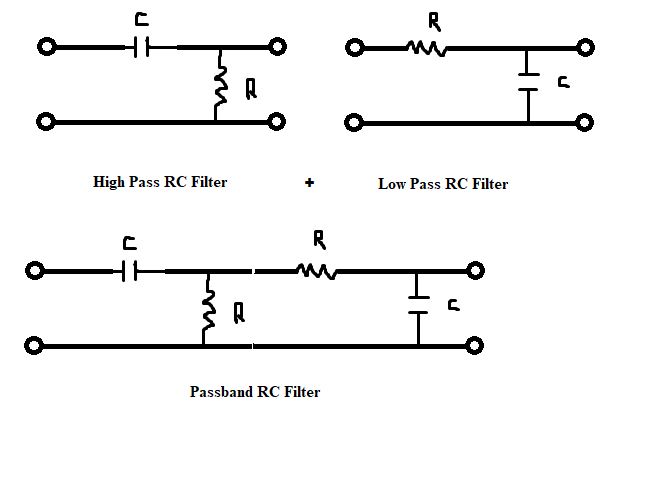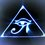# Passive Passband Filter

In my previous note on passive filters I showed how the passive first order low-pass RC filter and passive first order high-pass RC filter work. Now I will show how to make a passband filter and how the passband filter operates.In this case we will treat this as two separate devices each with their own cutoff frequency and each with their own attenuation factor. If you are unfamiliar with how to find the attenuation of a first order circuit make sure to check out my first note on passive filters where I describe the topic in detail.

$A_{v} = A_{v1} A_{v2}$

$A_{v}= \frac{R_{1}}{Z_{1}} \frac{X_{C2}}{Z_{2}}$

$A_{v}= (\frac{1}{\sqrt{1+(\frac{f_{c1}}{f})^{2}}} \angle \tan^{-1}(\frac{f_{c1}}{f}) )(\frac{1}{\sqrt{(\frac{f}{f_{c2}})^{2}+1}} \angle -\tan^{-1}(\frac{f}{f_{c2}}))$

$A_{v}=\frac{1}{\sqrt{(\frac{f}{f_{c2}})^{2}+1}\sqrt{1+(\frac{f_{c1}}{f})^{2}}}\angle \tan^{-1}(\frac{f_{c1}}{f})-\tan^{-1}(\frac{f}{f_{c2}})$

$A_{v}=\frac{1}{\sqrt{((\frac{f}{f_{c2}})^{2}+1)(1+(\frac{f_{c1}}{f})^{2}})}\angle \tan^{-1}(\frac{f_{c1}}{f})-\tan^{-1}(\frac{f}{f_{c2}})$

$A_{v}=\frac{1}{\sqrt{(\frac{f}{f_{c2}})^{2}+(\frac{f_{c1}}{f})^{2}+1+(\frac{f}{f_{c2}})^{2}(\frac{f_{c1}}{f})^{2}}}\angle \tan^{-1}(\frac{f_{c1}}{f})-\tan^{-1}(\frac{f}{f_{c2}})$

$A_{v}=\frac{1}{\sqrt{(\frac{f}{f_{c2}})^{2}+(\frac{f_{c1}}{f})^{2}+1+1}}\angle \tan^{-1}(\frac{f_{c1}}{f})-\tan^{-1}(\frac{f}{f_{c2}})$

$A_{v}=\frac{1}{\sqrt{(\frac{f}{f_{c2}})^{2}+(\frac{f_{c1}}{f})^{2}+2}}\angle \tan^{-1}(\frac{f_{c1}}{f})-\tan^{-1}(\frac{f}{f_{c2}})$

Note that $f_{c2}>f_{c1}$ and the greater the difference between the two the wider the pass band will be. If $f_{c2}=f_{c1}$ then there is no pass band because the minimum attenuation occurs at the highest attenuation factor when $f_{c2}=f_{c1}=f$ and results in an attenuation factor of $0.5$ which is greater than the desired minimum attenuation .

If we take the limit as $f_{c2}$ becomes infinitely larger than $f_{c1}$ then we reach the limit for the minimum attenuation (maximum attenuation factor = $\frac{1}{\sqrt{2}}$). Therefore this type of filter will always result in some attenuation.( If $f_{c2}$ is infinitely large then $\frac{f}{f_{c2}}$ approaches zero and if $f _{c1}$ is infinitely small then $\frac{f_{c1}}{f}$ approaches zero.)

Given that $f_{c2}>f_{c1}$ , there will be a frequency somewhere along the pass band called the resonant frequency which is where the maximum point of the graph will be. The frequency at which this occurs can be found by taking the geometric mean of the two cutoff frequencies (not the arithmetic mean).

The bandwidth of the filter is the difference between the two cutoff frequencies.

If the graph of attenuation vs frequency or voltage versus frequency is plotted so that the frequency axis has a logarithmic scale the curve will be symmetrical. If the frequency axis is scaled in the conventional method the curve will be asymmetrical. This is why the resonant frequency is found using the geometric mean and not the arithmetic mean.Note by Brody Acquilano
1 year, 11 months ago

This discussion board is a place to discuss our Daily Challenges and the math and science related to those challenges. Explanations are more than just a solution — they should explain the steps and thinking strategies that you used to obtain the solution. Comments should further the discussion of math and science.

When posting on Brilliant:

• Use the emojis to react to an explanation, whether you're congratulating a job well done , or just really confused .
• Ask specific questions about the challenge or the steps in somebody's explanation. Well-posed questions can add a lot to the discussion, but posting "I don't understand!" doesn't help anyone.
• Try to contribute something new to the discussion, whether it is an extension, generalization or other idea related to the challenge.

MarkdownAppears as
*italics* or _italics_ italics
**bold** or __bold__ bold
- bulleted- list
• bulleted
• list
1. numbered2. list
1. numbered
2. list
Note: you must add a full line of space before and after lists for them to show up correctly
paragraph 1paragraph 2

paragraph 1

paragraph 2

[example link](https://brilliant.org)example link
> This is a quote
This is a quote
    # I indented these lines
# 4 spaces, and now they show
# up as a code block.

print "hello world"
# I indented these lines
# 4 spaces, and now they show
# up as a code block.

print "hello world"
MathAppears as
Remember to wrap math in $$ ... $$ or $ ... $ to ensure proper formatting.
2 \times 3 $2 \times 3$
2^{34} $2^{34}$
a_{i-1} $a_{i-1}$
\frac{2}{3} $\frac{2}{3}$
\sqrt{2} $\sqrt{2}$
\sum_{i=1}^3 $\sum_{i=1}^3$
\sin \theta $\sin \theta$
\boxed{123} $\boxed{123}$